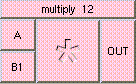MULTIPLYTHE BOX'S MAIN HELP: This is the multiplication function. The result is A * B. A and B can be scalars, vectors, or colors. The result will be a scalar if both A and B are scalars. The result will be a vector if either A or B is a vector. The result will be a color if either A or B is a color. A type mis-match will result from the multiplication of points and colors. A (input): This is the value to be multipied. The input may be a scalar, vector, or color. B (vinput): This is the value to be multipied. The input may be a scalar, vector, or color. OUT (output): This is the multiplication function. The result is A * B. A and B can be scalars, vectors, or colors. The result will be a scalar if both A and B are scalars. The result will be a vector if either A or B is a vector. The result will be a color if either A or B is a color. A type mis-match will result from the multiplication of points and colors.

<-- BACK TO Math